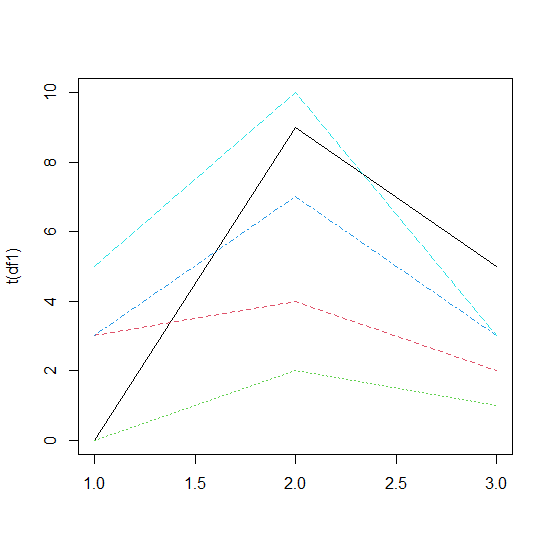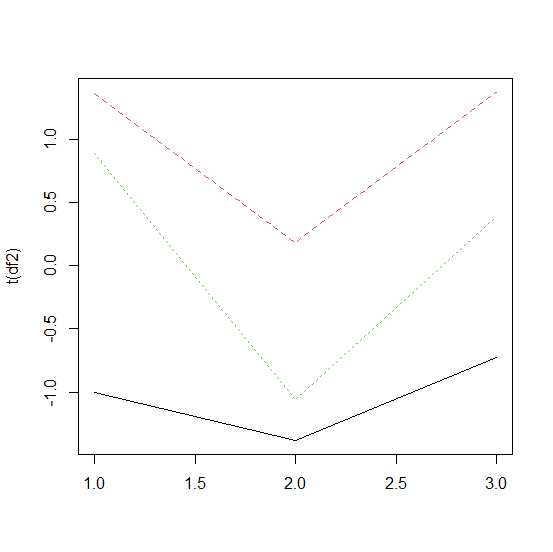# How to plot rows of a data frame as lines in R?

R ProgrammingServer Side ProgrammingProgramming

To plot row of a data frame as lines, we can use matplot function but we would need to transpose the data frame because transposed values of the data frame will be read as columns and the matplot function plot the columns not rows. For example, if we have a data frame called df then the plot of rows as lines can be created by using the command −

matplot(t(df),type="l")

## Example1

Consider the below data frame −

Live Demo

> x1<-rpois(5,2)
> x2<-rpois(5,5)
> x3<-rpois(5,3)
> df1<-data.frame(x1,x2,x3)
> df1

## Output

  x1 x2 x3
1  0  9  5
2  3  4  2
3  0  2  1
4  3  7  3
5  5 10  3

Creating plot of rows in df1 as lines −

> matplot(t(df1),type="l")

## Output## Example2

Live Demo

> y1<-rnorm(3)
> y2<-rnorm(3)
> y3<-rnorm(3)
> df2<-data.frame(y1,y2,y3)
> df2

## Output

          y1         y2         y3
1 -0.9992381 -1.3802480 -0.7228096
2  1.3677936  0.1813761  1.3711921
3  0.8905198 -1.0607813  0.3895616
1 -0.9992381 -1.3802480 -0.7228096
2  1.3677936  0.1813761  1.3711921
3  0.8905198 -1.0607813  0.3895616

Creating plot of rows in df2 as lines −

> matplot(t(df2),type="l")

## Output